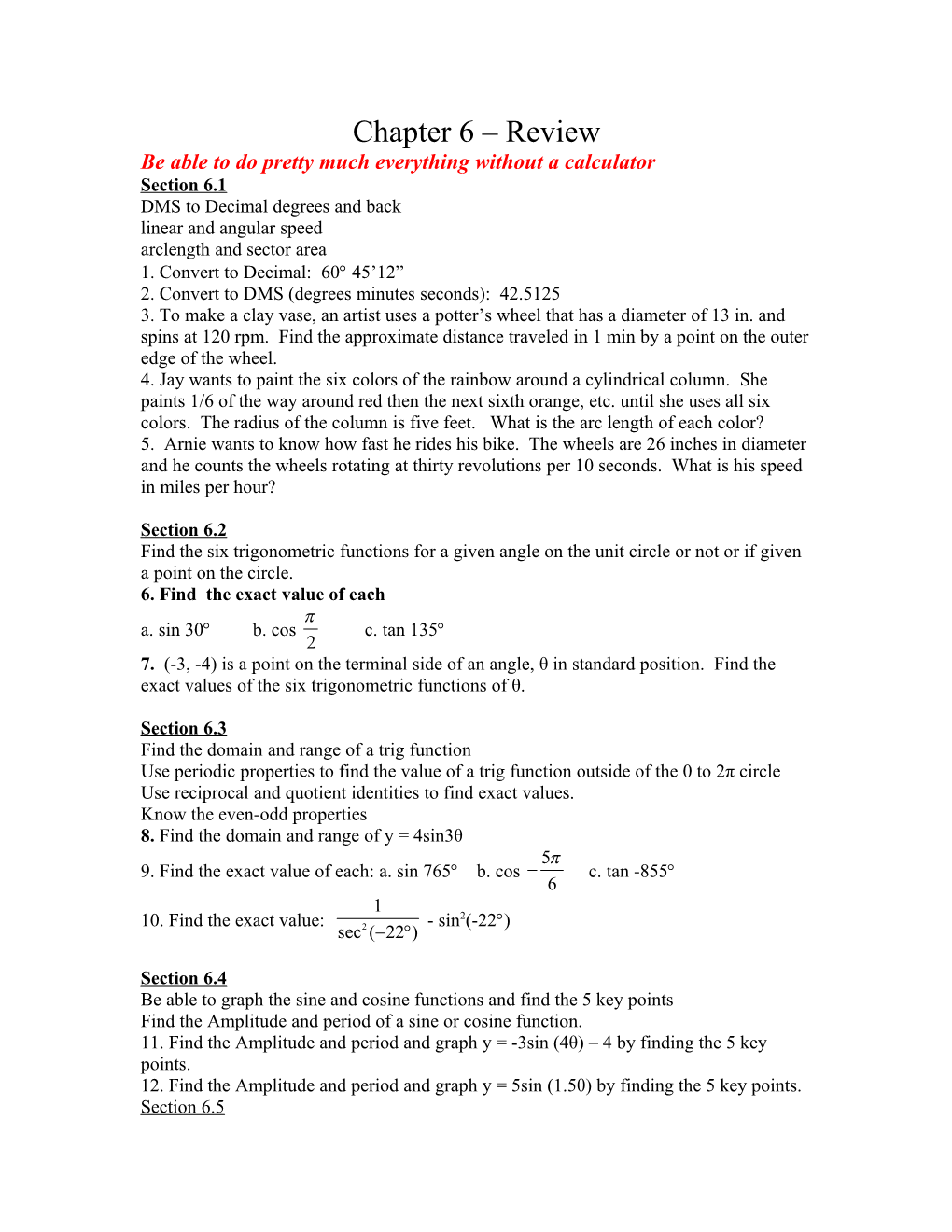# Be Able to Do Pretty Much Everything Without a CalculatorChapter 6 – Review

Be able to do pretty much everything without a calculator

Section 6.1

DMS to Decimal degrees and back

linear and angular speed

arclength and sector area

1. Convert to Decimal: 60 45’12”

2. Convert to DMS (degrees minutes seconds): 42.5125

3. To make a clay vase, an artist uses a potter’s wheel that has a diameter of 13 in. and spins at 120 rpm. Find the approximate distance traveled in 1 min by a point on the outer edge of the wheel.

4. Jay wants to paint the six colors of the rainbow around a cylindrical column. She paints 1/6 of the way around red then the next sixth orange, etc. until she uses all six colors. The radius of the column is five feet. What is the arclength of each color?

5. Arnie wants to know how fast he rides his bike. The wheels are 26 inches in diameter and he counts the wheels rotating at thirty revolutions per 10 seconds. What is his speed in miles per hour?

Section 6.2

Find the six trigonometric functions for a given angle on the unit circle or not or if given a point on the circle.

6. Find the exact value of each

a. sin 30b. cos c. tan 135

7. (-3, -4) is a point on the terminal side of an angle, θ in standard position. Find the exact values of the six trigonometric functions of θ.

Section 6.3

Find the domain and range of a trig function

Use periodic properties to find the value of a trig function outside of the 0 to 2π circle

Use reciprocal and quotient identities to find exact values.

Know the even-odd properties

8. Find the domain and range of y = 4sin3θ

9. Find the exact value of each: a. sin 765b. cos c. tan -855

10. Find the exact value: - sin2(-22)

Section 6.4

Be able to graph the sine and cosine functions and find the 5 key points

Find the Amplitude and period of a sine or cosine function.

11. Find the Amplitude and period and graph y = -3sin (4θ) – 4 by finding the 5 key points.

12. Find the Amplitude and period and graph y = 5sin (1.5θ) by finding the 5 key points.

Section 6.5

Be able to graph the remaining four functions. (tangent, cotangent, secant and cosecant)

13. Graph y = 7tan(3θ – π)

14. Graph y = ½ csc(θ) + 2

Section 6.6

Solve word problems involving Sinusoidal functions

Graph any sinusoidal function with a phase shift

Be able to build a sinusoidal function from data.

15. Find the amplitude, period and phase shift and graph

16. Model the data with a Sinusoidal function

Month, m / Average Monthly Temperature, T, in Phoenix, Arizona
Jan, 1 / 51
Feb, 2 / 55
Mar, 3 / 63
Apr, 4 / 67
May, 5 / 77
June, 6 / 86
Jul, 7 / 90
Aug, 8 / 90
Sep, 9 / 84
Oct, 10 / 71
Nov, 11 / 59
Dec,12 / 52